# Work Energy By Christos Work l Work is

• Slides: 18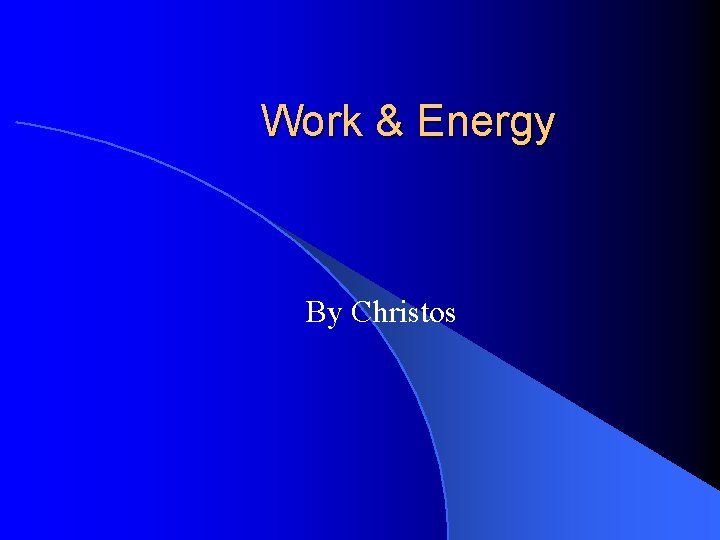Work & Energy By Christos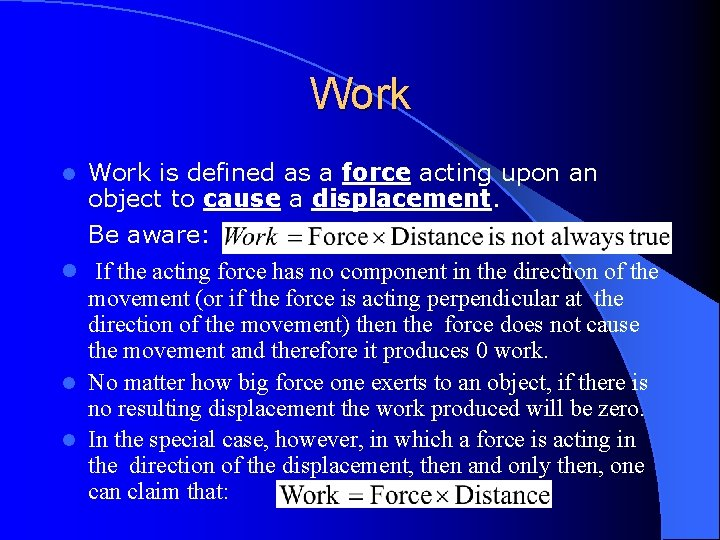Work l Work is defined as a force acting upon an object to cause a displacement. Be aware: l If the acting force has no component in the direction of the movement (or if the force is acting perpendicular at the direction of the movement) then the force does not cause the movement and therefore it produces 0 work. l No matter how big force one exerts to an object, if there is no resulting displacement the work produced will be zero. l In the special case, however, in which a force is acting in the direction of the displacement, then and only then, one can claim that: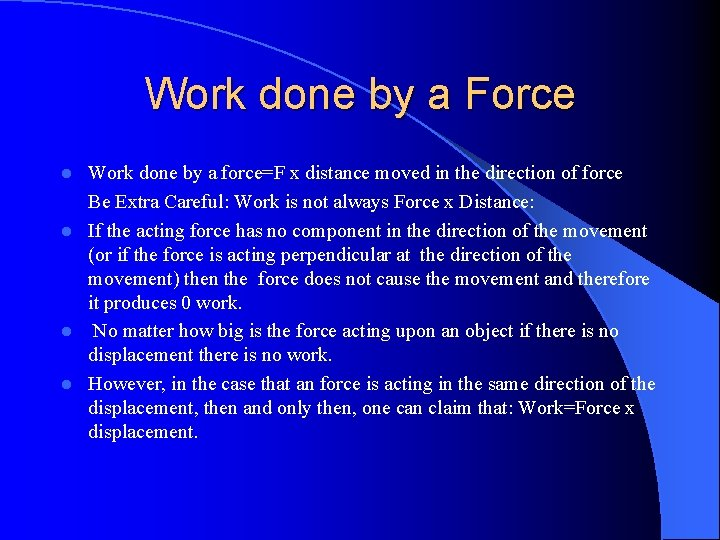Work done by a Force Work done by a force=F x distance moved in the direction of force Be Extra Careful: Work is not always Force x Distance: l If the acting force has no component in the direction of the movement (or if the force is acting perpendicular at the direction of the movement) then the force does not cause the movement and therefore it produces 0 work. l No matter how big is the force acting upon an object if there is no displacement there is no work. l However, in the case that an force is acting in the same direction of the displacement, then and only then, one can claim that: Work=Force x displacement. l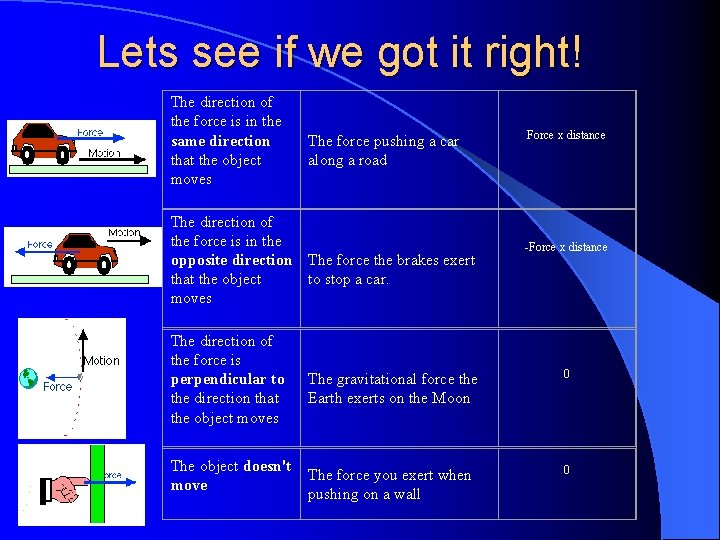Lets see if we got it right! The direction of the force is in the same direction that the object moves The force pushing a car along a road The direction of the force is in the opposite direction The force the brakes exert that the object to stop a car. moves The direction of the force is perpendicular to the direction that the object moves The object doesn't move Force x distance -Force x distance The gravitational force the Earth exerts on the Moon 0 The force you exert when pushing on a wall 0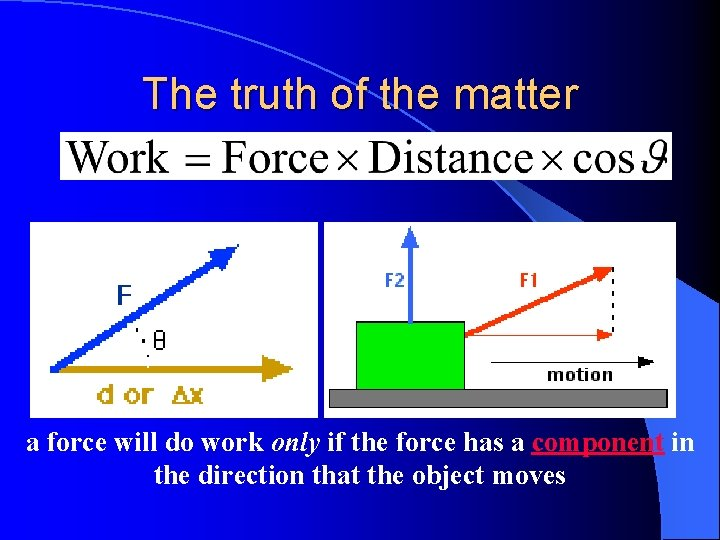The truth of the matter a force will do work only if the force has a component in the direction that the object moves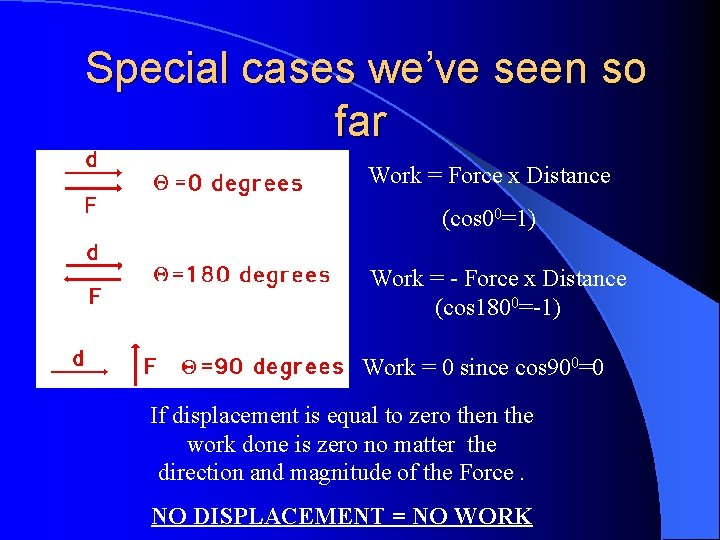Special cases we’ve seen so far Work = Force x Distance (cos 00=1) Work = - Force x Distance (cos 1800=-1) Work = 0 since cos 900=0 If displacement is equal to zero then the work done is zero no matter the direction and magnitude of the Force. NO DISPLACEMENT = NO WORK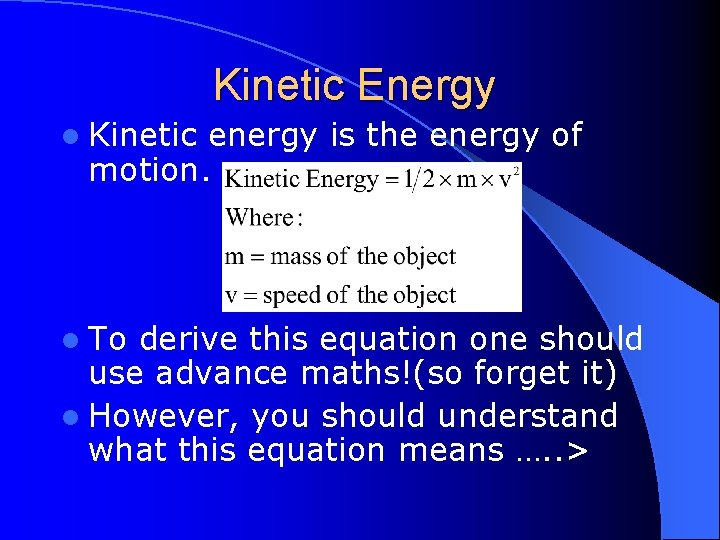Kinetic Energy l Kinetic energy is the energy of motion. l To derive this equation one should use advance maths!(so forget it) l However, you should understand what this equation means …. . >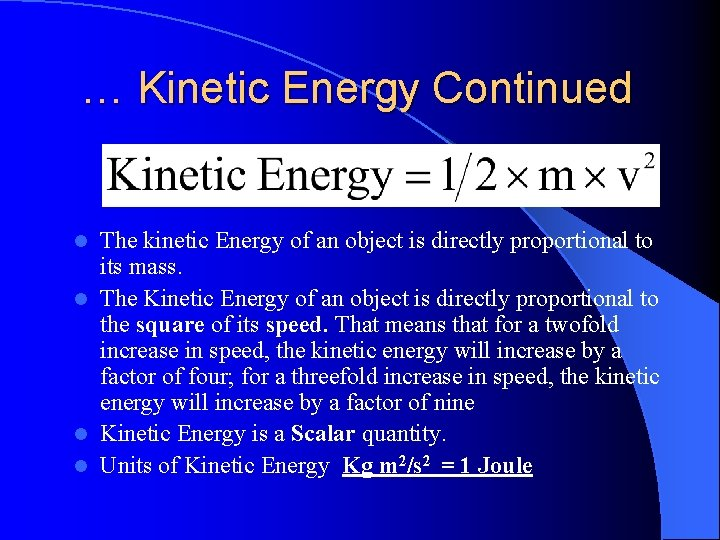… Kinetic Energy Continued The kinetic Energy of an object is directly proportional to its mass. l The Kinetic Energy of an object is directly proportional to the square of its speed. That means that for a twofold increase in speed, the kinetic energy will increase by a factor of four; for a threefold increase in speed, the kinetic energy will increase by a factor of nine l Kinetic Energy is a Scalar quantity. l Units of Kinetic Energy Kg m 2/s 2 = 1 Joule lLets check our understanding Suppose that you were captured by an evil physicist who gave you the following choice: You must either: l Stand in front of a 1000 kg. truck moving at 1 m/s, or l Stand in front of a 1 kg. meatball moving at 1000 m/s.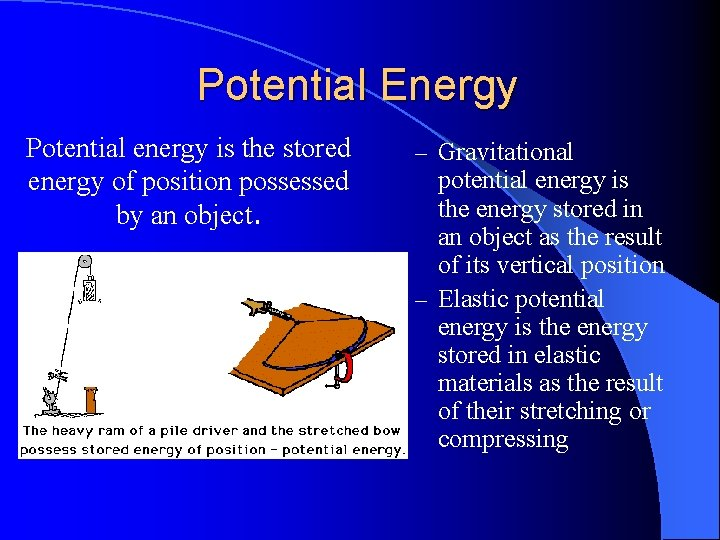Potential Energy Potential energy is the stored energy of position possessed by an object. – Gravitational potential energy is the energy stored in an object as the result of its vertical position – Elastic potential energy is the energy stored in elastic materials as the result of their stretching or compressing… Potential Energy Continued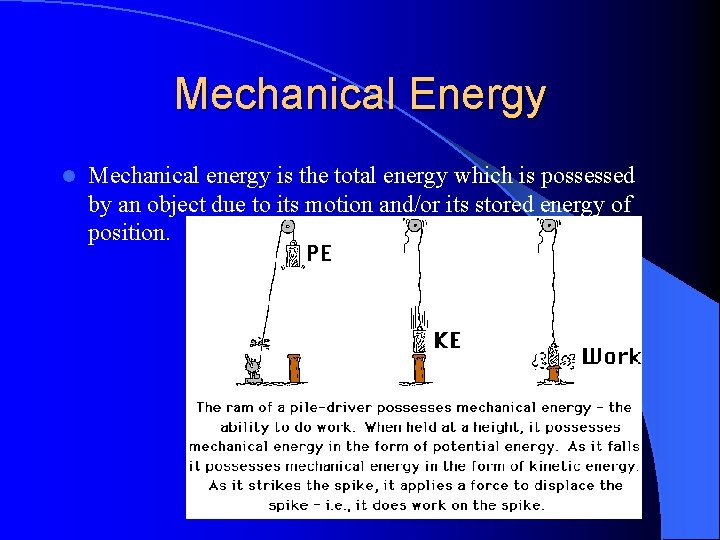Mechanical Energy l Mechanical energy is the total energy which is possessed by an object due to its motion and/or its stored energy of position.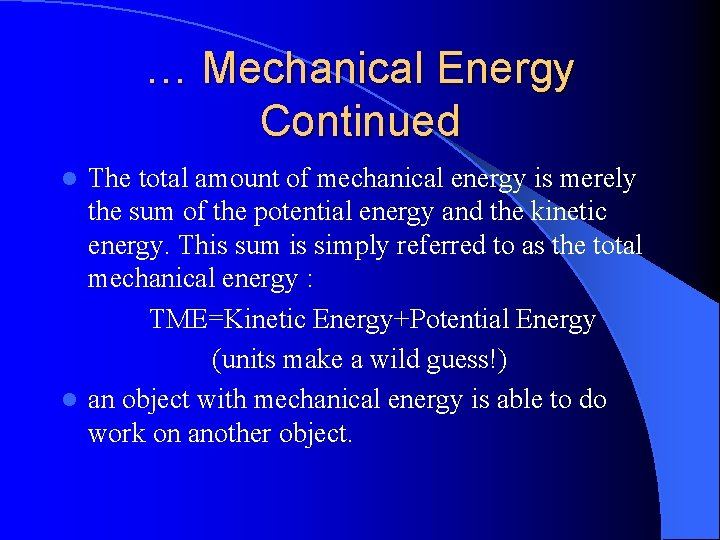… Mechanical Energy Continued The total amount of mechanical energy is merely the sum of the potential energy and the kinetic energy. This sum is simply referred to as the total mechanical energy : TME=Kinetic Energy+Potential Energy (units make a wild guess!) l an object with mechanical energy is able to do work on another object. l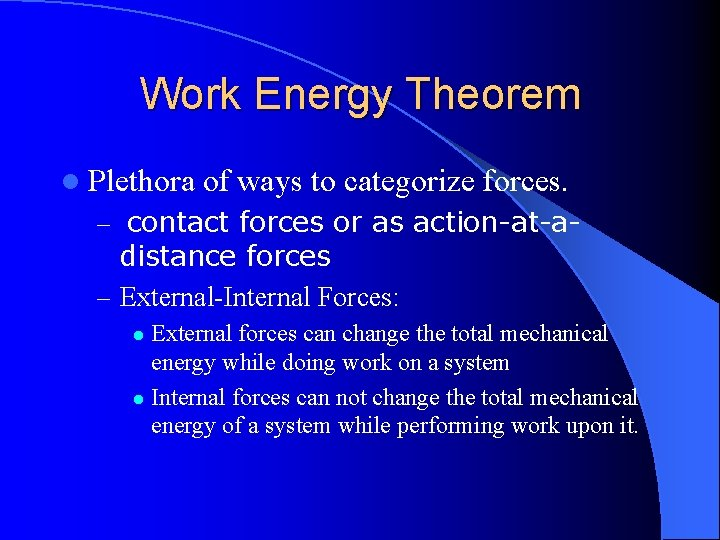Work Energy Theorem l Plethora of ways to categorize forces. – contact forces or as action-at-a- distance forces – External-Internal Forces: External forces can change the total mechanical energy while doing work on a system l Internal forces can not change the total mechanical energy of a system while performing work upon it. lExternal Forces applied forces, normal forces, tensional forces, friction forces, and air resistance forces. Work=change of mechanical energy l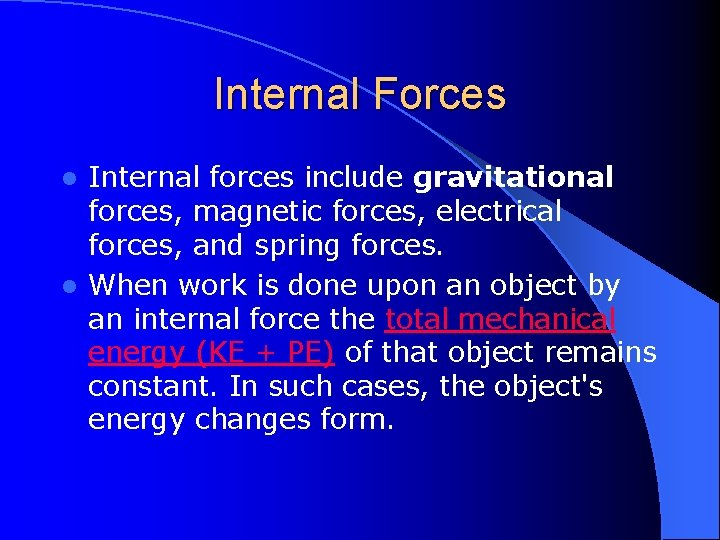Internal Forces Internal forces include gravitational forces, magnetic forces, electrical forces, and spring forces. l When work is done upon an object by an internal force the total mechanical energy (KE + PE) of that object remains constant. In such cases, the object's energy changes form. lThe roller coaster +Book http: //www. physicsclassroom. com/mmedia/qt/energy/coaster. mov http: //www. batesville. k 12. in. us/Physics/Phy. Net/Mechanics/Energy/lifting_a_book. htm http: //www. batesville. k 12. in. us/Physics/Phy. Net/Mechanics/Energy/pushing_a_bo ok. htm http: //www. batesville. k 12. in. us/Physics/Phy. Net/Mechanics/Energy/Work_as_Are a. htmlCheck our Understanding 1 Description of Motion A ball falls from a height of 2 meters in the absence of air resistance. 2 A skier glides from location A to location B across the friction free ice. 3 A baseball is travelling upward towards a man in the bleachers. A bungee chord begins to exert an upward force upon a falling bungee jumper. KE to PE or PE to KE? Explain.